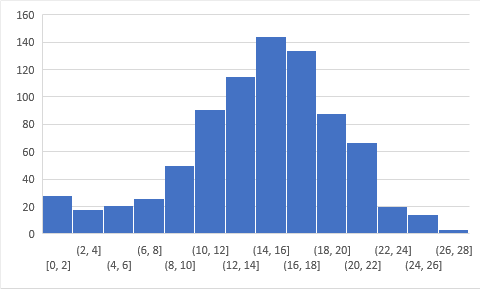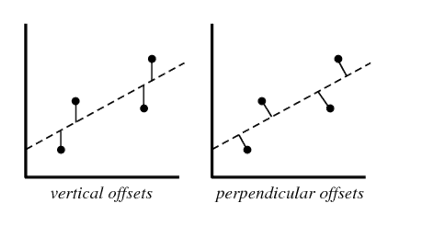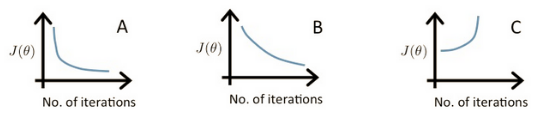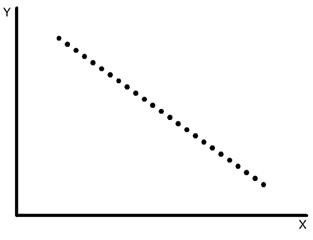Home » 30 Questions to test a data scientist on Linear Regression [Solution: Skilltest – Linear Regression]

# 30 Questions to test a data scientist on Linear Regression [Solution: Skilltest – Linear Regression]

## Introduction

Linear Regression is still the most prominently used statistical technique in data science industry and in academia to explain relationships between features.

A total of 1,355 people registered for this skill test. It was specially designed for you to test your knowledge on linear regression techniques. If you are one of those who missed out on this skill test, here are the questions and solutions. You missed on the real time test, but can read this article to find out how many could have answered correctly.

Here is the leaderboard for the participants who took the test.

## Overall Distribution

Below is the distribution of the scores of the participants:

##You can access the scores here. More than 800 people participated in the skill test and the highest score obtained was 28.

Here are some resources to get in depth knowledge in the subject.

Are you a beginner in Machine Learning? Do you want to master the concepts of Linear Regression and Machine Learning? Here is a beginner-friendly course to assist you in your journey –

## Skill test Questions and Answers

1) True-False: Linear Regression is a supervised machine learning algorithm.

A) TRUE
B) FALSE

Solution: (A)

Yes, Linear regression is a supervised learning algorithm because it uses true labels for training. Supervised learning algorithm should have input variable (x) and an output variable (Y) for each example.

2) True-False: Linear Regression is mainly used for Regression.

A) TRUE
B) FALSE

Solution: (A)

Linear Regression has dependent variables that have continuous values.

3) True-False: It is possible to design a Linear regression algorithm using a neural network?

A) TRUE
B) FALSE

Solution: (A)

True. A Neural network can be used as a universal approximator, so it can definitely implement a linear regression algorithm.

4) Which of the following methods do we use to find the best fit line for data in Linear Regression?

A) Least Square Error
B) Maximum Likelihood
C) Logarithmic Loss
D) Both A and B

Solution: (A)

In linear regression, we try to minimize the least square errors of the model to identify the line of best fit.

5) Which of the following evaluation metrics can be used to evaluate a model while modeling a continuous output variable?

A) AUC-ROC
B) Accuracy
C) Logloss
D) Mean-Squared-Error

Solution: (D)

Since linear regression gives output as continuous values, so in such case we use mean squared error metric to evaluate the model performance. Remaining options are use in case of a classification problem.

6) True-False: Lasso Regularization can be used for variable selection in Linear Regression.

A) TRUE
B) FALSE

Solution: (A)

True, In case of lasso regression we apply absolute penalty which makes some of the coefficients zero.

7) Which of the following is true about Residuals ?

A) Lower is better
B) Higher is better
C) A or B depend on the situation
D) None of these

Solution: (A)

Residuals refer to the error values of the model. Therefore lower residuals are desired.

8) Suppose that we have N independent variables (X1,X2… Xn) and dependent variable is Y. Now Imagine that you are applying linear regression by fitting the best fit line using least square error on this data.

You found that correlation coefficient for one of it’s variable(Say X1) with Y is -0.95.

Which of the following is true for X1?

A) Relation between the X1 and Y is weak
B) Relation between the X1 and Y is strong
C) Relation between the X1 and Y is neutral
D) Correlation can’t judge the relationship

Solution: (B)

The absolute value of the correlation coefficient denotes the strength of the relationship. Since  absolute correlation is very high it means that the relationship is strong between X1 and Y.

9) Looking at above two characteristics, which of the following option is the correct for Pearson correlation between V1 and V2?

If you are given the two variables V1 and V2 and they are following below two characteristics.

1. If V1 increases then V2 also increases

2. If V1 decreases then V2 behavior is unknown

A) Pearson correlation will be close to 1
B) Pearson correlation will be close to -1
C) Pearson correlation will be close to 0
D) None of these

Solution: (D)

We cannot comment on the correlation coefficient by using only statement 1.  We need to consider the both of these two statements. Consider V1 as x and V2 as |x|. The correlation coefficient would not be close to 1 in such a case.

10) Suppose Pearson correlation between V1 and V2 is zero. In such case, is it right to conclude that V1 and V2 do not have any relation between them?

A) TRUE
B) FALSE

Solution: (B)

Pearson correlation coefficient between 2 variables might be zero even when they have a relationship between them. If the correlation coefficient is zero, it just means that that they don’t move together. We can take examples like y=|x| or y=x^2.

11) Which of the following offsets, do we use in linear regression’s least square line fit? Suppose horizontal axis is independent variable and vertical axis is dependent variable.A) Vertical offset
B) Perpendicular offset
C) Both, depending on the situation
D) None of above

Solution: (A)

We always consider residuals as vertical offsets. We calculate the direct differences between actual value and the Y labels. Perpendicular offset are useful in case of PCA.

12) True- False: Overfitting is more likely when you have huge amount of data to train?

A) TRUE
B) FALSE

Solution: (B)

With a small training dataset, it’s easier to find a hypothesis to fit the training data exactly i.e. overfitting.

13) We can also compute the coefficient of linear regression with the help of an analytical method called “Normal Equation”. Which of the following is/are true about Normal Equation?

1. We don’t have to choose the learning rate
2. It becomes slow when number of features is very large
3. Thers is no need to iterate

A) 1 and 2
B) 1 and 3
C) 2 and 3
D) 1,2 and 3

Solution: (D)

14) Which of the following statement is true about sum of residuals of A and B?

Below graphs show two fitted regression lines (A & B) on randomly generated data. Now, I want to find the sum of residuals in both cases A and B.

Note:

1. Scale is same in both graphs for both axis.
2. X axis is independent variable and Y-axis is dependent variable.A) A has higher sum of residuals than B
B) A has lower sum of residual than B
C) Both have same sum of residuals
D) None of these

Solution: (C)

Sum of residuals will always be zero, therefore both have same sum of residuals

#### Question Context 15-17:

Suppose you have fitted a complex regression model on a dataset. Now, you are using Ridge regression with penality x.

15) Choose the option which describes bias in best manner.
A) In case of very large x; bias is low
B) In case of very large x; bias is high
C) We can’t say about bias
D) None of these

Solution: (B)

If the penalty is very large it means model is less complex, therefore the bias would be high.

16) What will happen when you apply very large penalty?

A) Some of the coefficient will become absolute zero
B) Some of the coefficient will approach zero but not absolute zero
C) Both A and B depending on the situation
D) None of these

Solution: (B)

In lasso some of the coefficient value become zero, but in case of Ridge, the coefficients become close to zero but not zero.

17) What will happen when you apply very large penalty in case of Lasso?
A) Some of the coefficient will become zero
B) Some of the coefficient will be approaching to zero but not absolute zero
C) Both A and B depending on the situation
D) None of these

Solution: (A)

As already discussed, lasso applies absolute penalty, so some of the coefficients will become zero.

18) Which of the following statement is true about outliers in Linear regression?

A) Linear regression is sensitive to outliers
B) Linear regression is not sensitive to outliers
C) Can’t say
D) None of these

Solution: (A)

The slope of the regression line will change due to outliers in most of the cases. So Linear Regression is sensitive to outliers.

19) Suppose you plotted a scatter plot between the residuals and predicted values in linear regression and you found that there is a relationship between them. Which of the following conclusion do you make about this situation?

A) Since the there is a relationship means our model is not good
B) Since the there is a relationship means our model is good
C) Can’t say
D) None of these

Solution: (A)

There should not be any relationship between predicted values and residuals. If there exists any relationship between them,it means that the model has not perfectly captured the information in the data.

#### Question Context 20-22:

Suppose that you have a dataset D1 and you design a linear regression model of degree 3 polynomial and you found that the training and testing error is “0” or in another terms it perfectly fits the data.

20) What will happen when you fit degree 4 polynomial in linear regression?
A) There are high chances that degree 4 polynomial will over fit the data
B) There are high chances that degree 4 polynomial will under fit the data
C) Can’t say
D) None of these

Solution: (A)

Since is more degree 4 will be more complex(overfit the data) than the degree 3 model so it will again perfectly fit the data. In such case training error will be zero but test error may not be zero.

21) What will happen when you fit degree 2 polynomial in linear regression?
A) It is high chances that degree 2 polynomial will over fit the data
B) It is high chances that degree 2 polynomial will under fit the data
C) Can’t say
D) None of these

Solution: (B)

If a degree 3 polynomial fits the data perfectly, it’s highly likely that a simpler model(degree 2 polynomial) might under fit the data.

22) In terms of bias and variance. Which of the following is true when you fit degree 2 polynomial?

A) Bias will be high, variance will be high
B) Bias will be low, variance will be high
C) Bias will be high, variance will be low
D) Bias will be low, variance will be low

Solution: (C)

Since a degree 2 polynomial will be less complex as compared to degree 3, the bias will be high and variance will be low.

Question Context 23:

Which of the following is true about below graphs(A,B, C left to right) between the cost function and Number of iterations?23) Suppose l1, l2 and l3 are the three learning rates for A,B,C respectively. Which of the following is true about l1,l2 and l3?

A) l2 < l1 < l3

B) l1 > l2 > l3
C) l1 = l2 = l3
D) None of these

Solution: (A)

In case of high learning rate, step will be high, the objective function will decrease quickly initially, but it will not find the global minima and objective function starts increasing after a few iterations.

In case of low learning rate, the step will be small. So the objective function will decrease slowly

Question Context 24-25:

We have been given a dataset with n records in which we have input attribute as x and output attribute as y. Suppose we use a linear regression method to model this data. To test our linear regressor, we split the data in training set and test set randomly.

24) Now we increase the training set size gradually. As the training set size increases, what do you expect will happen with the mean training error?

A) Increase
B) Decrease
C) Remain constant
D) Can’t Say

Solution: (D)

Training error may increase or decrease depending on the values that are used to fit the model. If the values used to train contain more outliers gradually, then the error might just increase.

25) What do you expect will happen with bias and variance as you increase the size of training data?

A) Bias increases and Variance increases
B) Bias decreases and Variance increases
C) Bias decreases and Variance decreases
D) Bias increases and Variance decreases
E) Can’t Say False

Solution: (D)

As we increase the size of the training data, the bias would increase while the variance would decrease.

Question Context 26:

Consider the following data where one input(X) and one output(Y) is given.26) What would be the root mean square training error for this data if you run a Linear Regression model of the form (Y = A0+A1X)?

A) Less than 0
B) Greater than zero
C) Equal to 0
D) None of these

Solution: (C)

We can perfectly fit the line on the following data so mean error will be zero.

Question Context 27-28:

Suppose you have been given the following scenario for training and validation error for Linear Regression.

 Scenario Learning Rate Number of iterations Training Error Validation Error 1 0.1 1000 100 110 2 0.2 600 90 105 3 0.3 400 110 110 4 0.4 300 120 130 5 0.4 250 130 150

27) Which of the following scenario would give you the right hyper parameter?

A) 1
B) 2
C) 3
D) 4

Solution: (B)

Option B would be the better option because it leads to less training as well as validation error.

28) Suppose you got the tuned hyper parameters from the previous question. Now, Imagine you want to add a variable in variable space such that this added feature is important. Which of the following thing would you observe in such case?

A) Training Error will decrease and Validation error will increase

B) Training Error will increase and Validation error will increase
C) Training Error will increase and Validation error will decrease
D) Training Error will decrease and Validation error will decrease
E) None of the above

Solution: (D)

If the added feature is important, the training and validation error would decrease.

Question Context 29-30:

Suppose, you got a situation where you find that your linear regression model is under fitting the data.

29) In such situation which of the following options would you consider?

2. Start introducing polynomial degree variables
3. Remove some variables

A) 1 and 2
B) 2 and 3
C) 1 and 3
D) 1, 2 and 3

Solution: (A)

In case of under fitting, you need to induce more variables in variable space or you can add some polynomial degree variables to make the model more complex to be able to fir the data better.

30) Now situation is same as written in previous question(under fitting).Which of following regularization algorithm would you prefer?

A) L1
B) L2
C) Any
D) None of these

Solution: (D)

I won’t use any regularization methods because regularization is used in case of overfitting.

## End Notes

I tried my best to make the solutions as comprehensive as possible but if you have any questions / doubts please drop in your comments below. I would love to hear your feedback about the skilltest. For more such skilltests, check out our current hackathons.

You can also read this article on our Mobile APP•mej says:

For question 4, isn’t (D) the right answer? Can’t we use OLS or MLE to find best fit line in Linear Regression? I had thought MLE would be better for complex data.

•Mateusz says:

7) Which of the following is true about Residuals ?

A) Lower is better
B) Higher is better
C) A or B depend on the situation
D) None of these

The correct answer is D. Lower Residuals SQUARES are better than higher residuals squares!

•Asw says:

A good place to test yourself ! Great effort! Really helped.

•Shweta says:

Hey Ankit

Thanks for all these questions.
If possible can you please post more question on Linear as well as Multiple regression and on Hypothesis theory as well.

•Magdalena Deschner says: